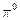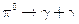Chapter 33, Problem 19PE

Chapter
Section
Textbook Problem

(a) What is the uncertainty in the energy released in the decay of adue to its short lifetime?(b) What traction of the decay energy is this, noting that the decay mode is(so that all themass is destroyed)?

To determine

(a)

The uncertainty in the energy released in the decay of a π0 due to its short life time.

Explanation

Given:

π0 particle is having short life time.

Formula used:

The uncertainty principle for energy and time is given by, ΔE.Δt=h4π

Where, ΔE uncertainty in the measurement of energy

Δt uncertainty in the measurement of time

Calculation:

Life time of π0 particle =8.4×1017 s

Therefore, uncertainty in the energy released, ΔE=h4πΔt

=6

To determine

(b)

What fraction of decay energy is the amount of uncertainty in the energy released.

Still sussing out bartleby?

Check out a sample textbook solution.

See a sample solution

The Solution to Your Study Problems

Bartleby provides explanations to thousands of textbook problems written by our experts, many with advanced degrees!

Get Started